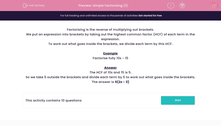# Simple Factorising (1)

In this worksheet, students factorise simple algebraic expressions.Key stage:  KS 2

Curriculum topic:   Maths and Numerical Reasoning

Curriculum subtopic:   Equations and Algebra

Difficulty level:#### Worksheet Overview

Factorising is the reverse of multiplying out brackets.

We put an expression into brackets by taking out the highest common factor (HCF) of each term in the expression.

To work out what goes inside the brackets, we divide each term by this HCF.

Example

Factorise fully 10x - 15

The HCF of 10x and 15 is 5.

So we take 5 outside the brackets and divide each term by 5 to work out what goes inside the brackets.

The answer is 5(2x - 3)

### What is EdPlace?

We're your National Curriculum aligned online education content provider helping each child succeed in English, maths and science from year 1 to GCSE. With an EdPlace account you’ll be able to track and measure progress, helping each child achieve their best. We build confidence and attainment by personalising each child’s learning at a level that suits them.

Get started